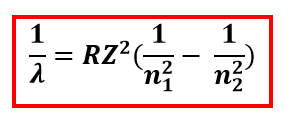Chemistry Practice Problems Bohr and Balmer Equations Practice Problems Solution: Why are the emission wavelengths for hydrogen and ...

# Solution: Why are the emission wavelengths for hydrogen and helium different? And why does the equation that relates to emission spectra to the energy levels differ for hydrogen and helium?

###### Problem

Why are the emission wavelengths for hydrogen and helium different? And why does the equation that relates to emission spectra to the energy levels differ for hydrogen and helium?

###### Solution

The Rydberg equation relates the emission wavelength to the energy levels:whereView Complete Written Solution

Bohr and Balmer Equations

Bohr and Balmer Equations

#### Q. What is the wavelength of the line corresponding to n=5 in the Balmer series?

Solved • Thu Aug 29 2019 14:25:49 GMT-0400 (EDT)

Bohr and Balmer Equations

#### Q. What is the wavelength of the line corresponding to n=4 in the Balmer series?

Solved • Thu Aug 29 2019 14:22:59 GMT-0400 (EDT)

Bohr and Balmer Equations

#### Q. Calculate the energy of a photon emitted when an electron in a hydrogen atom relaxes from n = 5 to n = 3?

Solved • Thu Aug 08 2019 01:11:10 GMT-0400 (EDT)

Bohr and Balmer Equations

#### Q. Calculate the wavelength of light emitted when the electron in the hydrogen atom undergoes a transition from level n4 to n3?

Solved • Wed Aug 07 2019 22:48:42 GMT-0400 (EDT)